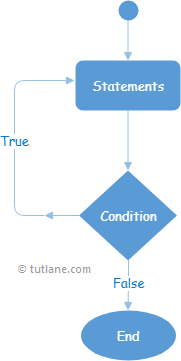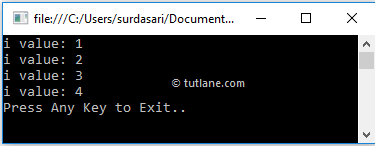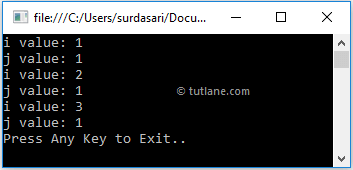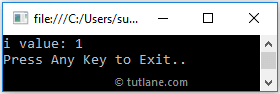# C# Do While Loop with Examples

In c#, the Do-While loop is used to execute a block of statements until the specified expression return as true.

Generally, in c# the do-while loop is same as the while loop, but only the difference is while loop will execute the statements only when the defined condition returns true, but the do-while loop will execute the statements at least once because first it will execute the block of statements and then it will checks the condition.

## Syntax of C# Do-While Loop

Generally, do and while keywords are used to create a do...while loop in C#. Following is the syntax of defining a do-while loop in c# programming language to execute the block of statements until the defined condition evaluates as false.

do
{

// Statements to Execute

}while (boolean_expression);

If you observe the above syntax, the do-while loop starts with the do keyword followed by a block of statements and while with a parameter called boolean_expression.

Here the body of the do-while loop will be executed first, and the boolean_expression will be evaluated. If boolean_expression returns true again, the statements inside of the do-while loop will be executed.

In case the boolean_expression is evaluated to false, then the do-while loop stops the execution of statements, and the program comes out of the loop.

## C# Do…While Loop Flow Chart Diagram

Following is the pictorial representation of the do-while loop process flow in the c# programming language.Now we will see how to use the do-while loop in the c# programming language with examples.

## C# Do…While Loop Example

Following is the example of using a do-while loop in c# programming language to execute the block of statements based on our requirements.

using System;

namespace Tutlane
{
class Program
{
static void Main(string[] args)
{
int i = 1;
do
{
Console.WriteLine("i value: {0}", i);
i++;
} while (i <= 4);
Console.WriteLine("Press Enter Key to Exit..");
}
}
}

If you observe the above example, first we are executing the statements within the do-while loop and increasing the variable i (i++) value to 1 by using the increment operator.

After that, the condition (i <= 4) will be evaluated, and again it will execute the block of statements if the condition returns true otherwise, it terminates the loop.

When we execute the above c# program, we will get the result as shown below.If you observe the above result, the do-while loop has been executed until it matches the defined condition (i <= 4), and the program came out of the loop whenever the defined condition returns false.

## C# Nested Do-While Loop

In c#, we can use one do-while loop within another do-while loop to implement the application based on our requirements.

Following is the example of implementing a nested do-while loop in the c# programming language.

using System;

namespace Tutlane
{
class Program
{
static void Main(string[] args)
{
int i = 1;
do
{
Console.WriteLine("i value: {0}", i);
i++;
int j = 1;
do
{
Console.WriteLine("j value: {0}", j);
j++;
} while (j < 2);
} while (i < 4);
Console.WriteLine("Press Enter Key to Exit..");
}
}
}

If you observe the above example, we used one do-while loop within another do-while loop to achieve nested do-while loop functionality in our application based on our requirements.

When we execute the above c# program, we will get the result as shown below.If you observe the above example, both do-while loops got executed and returned the result based on our requirements.

## C# Do-While Loop with Break Statement

In c#, we can exit or terminate the execution of a do-while loop immediately by using the break keyword.

Following is the example of using the break keyword in a do-while loop to terminate the loop's execution in the c# programming language.

using System;

namespace Tutlane
{
class Program
{
static void Main(string[] args)
{
int i = 1;
do
{
Console.WriteLine("i value: {0}", i);
i++;
if (i == 2)
break;
} while(i < 4);
Console.WriteLine("Press Enter Key to Exit..");
}
}
}

If you observe the above example, whenever the variable (i) value becomes 2, we terminate the loop using the break statement.

When we execute the above c# program, we will get the result as shown below.This is how we can use the break statement with a do-while loop to terminate the loop's execution based on our requirements.